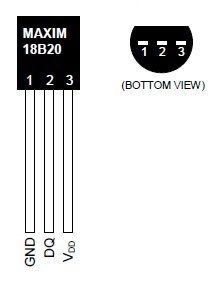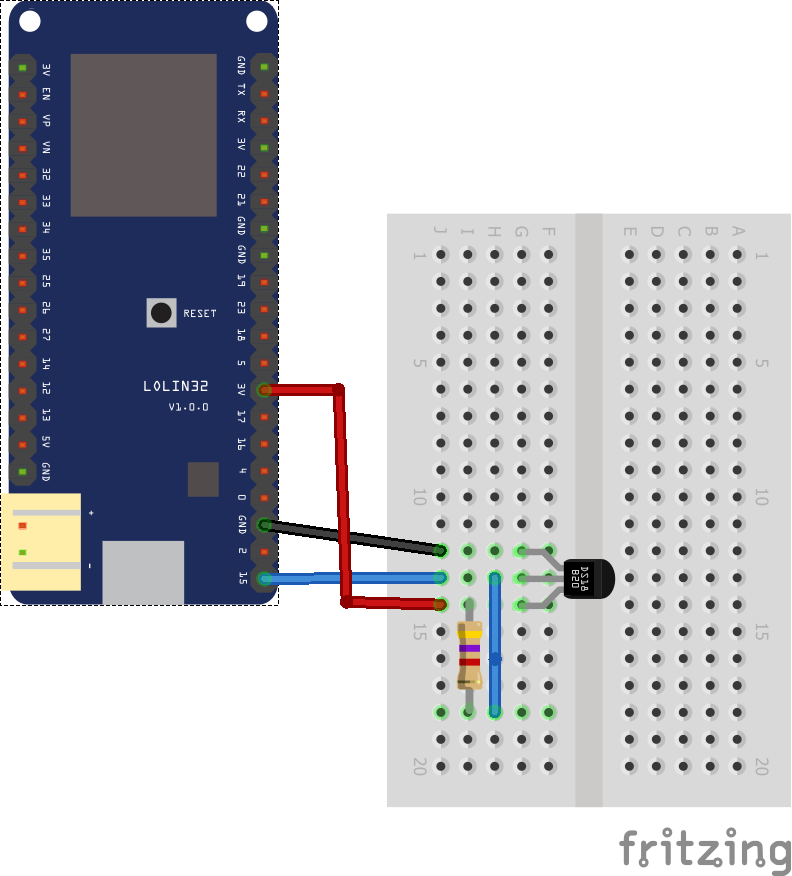Home » ESP32 and DS18B20 temperature sensor example

# ESP32 and DS18B20 temperature sensor example

The DS18B20 is a  temperature sensor that can be used in various simple projects.  This part uses the 1 wire (I2C) bus and you can connect multiple sensors up to your Arduino.

The part is also relatively low cost and only requires an additional 4k7 pull up resistor. In the example below we shall make a basic example that reads the temperature and outputs via serial and can be verified using the serial monitor in the Arduino IDE.

Lets look at the parts list

Parts List

Label Part Type
DS1 DS18B20 1-Wire Temperature Sensor
Part1 LOLIN32 (Rev3)
R1 4.7k Ω Resistor

Layout

As always be careful not to get the connections incorrect, you can refer to the pinout for the device below to help . The DS18B20 can be powered by between 3.0V and 5.5V so you can simply connect its GND pin to 0V and the VDD pin to +5V from the Wemos Minids18b20 pinout

Here is the connection diagram showing how to connect your wemos to the resistor and sensor.

Schematicesp32 and ds18b20

Code

[cpp]

#include <OneWire.h>

// OneWire DS18S20, DS18B20, DS1822 Temperature Example

OneWire ds(A13); // on pin D4 (a 4.7K resistor is necessary)

void setup(void)
{
Serial.begin(9600);
}

void loop(void)
{
byte i;
byte present = 0;
byte type_s;
byte data;
float celsius, fahrenheit;

{
ds.reset_search();
delay(250);
return;
}

{
Serial.println(“CRC is not valid!”);
return;
}
//Serial.println();

// the first ROM byte indicates which chip
{
case 0x10:
type_s = 1;
break;
case 0x28:
type_s = 0;
break;
case 0x22:
type_s = 0;
break;
default:
Serial.println(“Device is not a DS18x20 family device.”);
return;
}

ds.reset();
ds.write(0x44, 1); // start conversion, with parasite power on at the end
delay(1000);
present = ds.reset();

for ( i = 0; i < 9; i++)
{
}

// Convert the data to actual temperature
int16_t raw = (data << 8) | data;
if (type_s) {
raw = raw << 3; // 9 bit resolution default
if (data == 0x10)
{
raw = (raw & 0xFFF0) + 12 – data;
}
}
else
{
byte cfg = (data & 0x60);
if (cfg == 0x00) raw = raw & ~7; // 9 bit resolution, 93.75 ms
else if (cfg == 0x20) raw = raw & ~3; // 10 bit res, 187.5 ms
else if (cfg == 0x40) raw = raw & ~1; // 11 bit res, 375 ms

}
celsius = (float)raw / 16.0;
fahrenheit = celsius * 1.8 + 32.0;
Serial.print(“Temperature = “);
Serial.print(celsius);
Serial.print(” Celsius, “);
Serial.print(fahrenheit);
Serial.println(” Fahrenheit”);
}

[/cpp]

Output

Open the serial monitor and you should see something like this

Temperature = 20.62 Celsius, 69.12 Fahrenheit
Temperature = 20.81 Celsius, 69.46 Fahrenheit
Temperature = 22.12 Celsius, 71.82 Fahrenheit
Temperature = 23.12 Celsius, 73.62 Fahrenheit
Temperature = 23.87 Celsius, 74.97 Fahrenheit
Temperature = 24.44 Celsius, 75.99 Fahrenheit
Temperature = 24.81 Celsius, 76.66 Fahrenheit
Temperature = 25.19 Celsius, 77.34 Fahrenheit
Temperature = 25.44 Celsius, 77.79 Fahrenheit
Temperature = 25.75 Celsius, 78.35 Fahrenheit
Temperature = 25.94 Celsius, 78.69 Fahrenheit